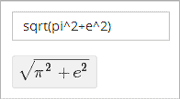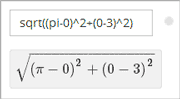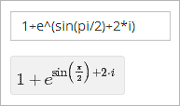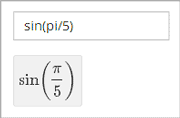# 12. Entering Mathematical and Scientific Expressions¶

For some math, science, and other problems, you’ll enter a numerical or math expression, such as a formula, into a response field. You enter your response as plain text, and the edX system then converts your text into numbers and symbols that appear below the response field:Additionally, some edX courses have a built-in calculator tool that you can use while you work through the course. If the course has a calculator, the calculator appears as a small icon in the lower-right corner of all pages in the courseware.When you select the icon, the calculator opens along the lower edge of the browser window. You can close the calculator by selecting the X just above the upper-right corner of the calculator.Both the calculator and the response fields in math problems accept a selection of characters that represent numbers, operators, constants, functions, and other mathematical concepts. You may recognize parts of this system if you’ve used math programs before.

Note

You can select the circled `i` icon on the right side of the calculator input field to see a pop-up hint that has an abbreviated list of these characters.

## 12.1. Entering Math Expressions¶

When you enter your plain text into the calculator or the response field, follow these guidelines:

• Arithmetical operations: Use standard characters for addition (+), subtraction (-), multiplication (*), and division (/).
• Multiplication: Be sure to indicate multiplication explicitly. That is, instead of `mc^2` type `m*c^2`, and instead of `5a+4b+3c` type `5*a+4*b+3*c`.
• Operation order: Use parentheses (`( )`) to specify the order of operations and to make your expression as clear as possible. Use curved parentheses (`( )`) only. Do not use brackets (`[ ]`) or braces (```{ }```).
• Exponents or superscripts: Insert a caret (^) before an exponent or superscript. If the exponent or superscript includes multiple characters or is an expression, surround the expression with parentheses. For example, you can enter `x^n` or `x^(n-1)`.
• Subscripts: Insert an underscore (_) before a subscript to indicate a subscript. If the subscript has multiple characters, type the characters without a space. For example, you can enter `v_IN-v_OUT`. Note, however, that subscripts cannot currently include operators or parentheses.
• Avoid whitespace.
• Don’t use equal signs (=).
• Because the system is case-sensitive, make sure you use uppercase and lowercase letters carefully.

Note

The edX system accepts both constants and metric affixes. Be careful to distinguish between constants and metric affixes. Constants stand alone, while metric affixes must be combined with numbers.

For example, `c` can be a constant representing the speed of light or a metric affix meaning “centi”. When you use `c` as a metric affix, don’t include a space between `c` and the number. When you use `c` as a constant, indicate multiplication explicitly. The following examples show the difference:

• `2c` = `0.02` (2 multiplied by 0.01)
• `2*c` = `599584916.0` (the speed of light multiplied by 2)
• `2M` = `2,000,000` (2 multiplied by 1,000,000)
• `2*M` = 2 multiplied by the variable M

### 12.1.1. Numbers¶

You can use the following types of numbers:

• Integers: 2520
• Fractions: 2/3
• Normal floats: 3.14
• Floats with no integer part: .98

The largest number you can use is 1.7977e+308, which is the largest float possible in the Python programming language.

### 12.1.2. Scientific Notation and Metric Affixes¶

You can enter metric affixes or scientific notation to indicate very large or very small numbers. For scientific notation, you can type either a caret (^) or the letter `e` followed by a number to indicate an exponent. You can use both positive and negative exponents.

For example, to indicate `0.012`, you can enter either of the following expressions:

• `1.2^-2`
• `1.2e-2`

To indicate `-440,000`, you can enter either of the following expressions:

• `-4.4^5`
• `-4.4e5`

The following table shows how to enter numbers with metric affixes, with scientific notation, and with `e` notation.

To enter this number Use this metric affix Use this scientific notation Use this `e` notation Other notation
0.1 1d (deci) 10^-1 1e-1
0.01 1c (centi) 10^-2 1e-2 1% (percent)
0.001 1m (milli) 10^-3 1e-3
0.000001 1u (micro) 10^-6 1e-6
0.000000001 1n (nano) 10^-9 1e-9
0.000000000001 1p (pico) 10^-12 1e-12
1000 1k (kilo) 10^3 1e3
1,000,000 1M (mega) 10^6 1e6
1,000,000,000 1G (giga) 10^9 1e9
1,000,000,000,000 1T (tera) 10^12 1e12

Note

When you use metric affixes or `e` notation, make sure you do not include spaces between the number and the metric affix or the `e`.

### 12.1.3. Constants¶

You can use several different constants in your mathematical expressions.

Note

When you enter constants multiplied by a number, make sure to indicate the multiplication explicitly. For example, enter `2*c` instead of `2c` and `-4*i` instead of `-4i`.

Constant Value
`c` The speed of light in m/s (2.998^8)
`e` Euler’s number (2.718...)
`g` Gravity (9.80 m/s^2)
`i` The square root of -1
`j` The square root of -1
`k` The Boltzmann constant (~1.38^-23 in Joules/Kelvin)
`pi` The ratio of a circle’s circumference to its diameter (3.14159...)
`q` The fundamental charge (~1.602^-19 Coloumbs)
`T` The positive difference between 0K and 0°C (273.15)

### 12.1.4. Greek Letters¶

To use any of the following Greek letters, type the name of the letter in the calculator or the response field.

 alpha beta gamma delta epsilon varepsilon zeta eta theta vartheta iota kappa lambda mu nu xi pi rho sigma tau upsilon phi varphi chi psi omega

Note

`epsilon` is the lunate version, whereas `varepsilon` looks like a backward 3.

### 12.1.5. Functions¶

To use a function, type the letters that represent the function, and then surround the expression in that function with parentheses. For example, to represent the square root of `4*a+b`, type `sqrt(4*a+b)`.

You can use the following functions:

• Common functions
• sqrt
• log10
• log2
• ln
• exp
• abs
• Trigonometric functions and their inverses
• sin
• cos
• tan
• sec
• csc
• cot
• arcsin
• arccos
• arctan
• arcsec
• arccsc
• arccot
• Hyperbolic trigonometric functions and their inverses
• sinh
• cosh
• tanh
• sech
• csch
• coth
• arcsinh
• arccosh
• arctanh
• arcsech
• arccsch
• arccoth
• Factorials: Enter factorials as `fact(3)` or `factorial(3)`. You must use integers. For example, you can’t enter `fact(1.5)`.
• A “parallel resistors” operator (`||`). For example, `1 || 2` represents the resistance of a pair of parallel resistors (of resistance 1 and 2 ohms), evaluating to 2/3 (ohms).